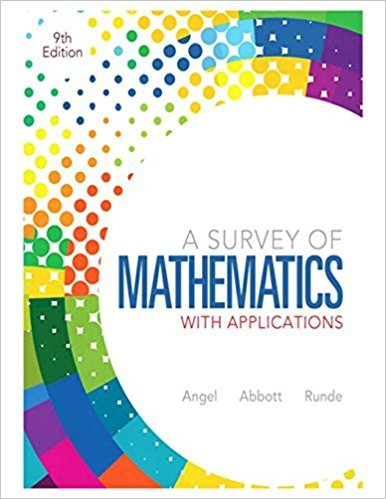×
×

# Solutions for Chapter 11.4: Consumer Mathematics## Full solutions for A Survey of Mathematics with Applications | 9th Edition

ISBN: 9780321759665Solutions for Chapter 11.4: Consumer Mathematics

Solutions for Chapter 11.4
4 5 0 303 Reviews
25
1
##### ISBN: 9780321759665

This expansive textbook survival guide covers the following chapters and their solutions. This textbook survival guide was created for the textbook: A Survey of Mathematics with Applications, edition: 9. A Survey of Mathematics with Applications was written by and is associated to the ISBN: 9780321759665. Chapter 11.4: Consumer Mathematics includes 43 full step-by-step solutions. Since 43 problems in chapter 11.4: Consumer Mathematics have been answered, more than 70460 students have viewed full step-by-step solutions from this chapter.

Key Math Terms and definitions covered in this textbook
• Big formula for n by n determinants.

Det(A) is a sum of n! terms. For each term: Multiply one entry from each row and column of A: rows in order 1, ... , nand column order given by a permutation P. Each of the n! P 's has a + or - sign.

• Block matrix.

A matrix can be partitioned into matrix blocks, by cuts between rows and/or between columns. Block multiplication ofAB is allowed if the block shapes permit.

• Free variable Xi.

Column i has no pivot in elimination. We can give the n - r free variables any values, then Ax = b determines the r pivot variables (if solvable!).

• Gauss-Jordan method.

Invert A by row operations on [A I] to reach [I A-I].

• Hankel matrix H.

Constant along each antidiagonal; hij depends on i + j.

• Incidence matrix of a directed graph.

The m by n edge-node incidence matrix has a row for each edge (node i to node j), with entries -1 and 1 in columns i and j .

• Independent vectors VI, .. " vk.

No combination cl VI + ... + qVk = zero vector unless all ci = O. If the v's are the columns of A, the only solution to Ax = 0 is x = o.

• Krylov subspace Kj(A, b).

The subspace spanned by b, Ab, ... , Aj-Ib. Numerical methods approximate A -I b by x j with residual b - Ax j in this subspace. A good basis for K j requires only multiplication by A at each step.

• lA-II = l/lAI and IATI = IAI.

The big formula for det(A) has a sum of n! terms, the cofactor formula uses determinants of size n - 1, volume of box = I det( A) I.

• Nilpotent matrix N.

Some power of N is the zero matrix, N k = o. The only eigenvalue is A = 0 (repeated n times). Examples: triangular matrices with zero diagonal.

• Normal equation AT Ax = ATb.

Gives the least squares solution to Ax = b if A has full rank n (independent columns). The equation says that (columns of A)·(b - Ax) = o.

• Partial pivoting.

In each column, choose the largest available pivot to control roundoff; all multipliers have leij I < 1. See condition number.

• Permutation matrix P.

There are n! orders of 1, ... , n. The n! P 's have the rows of I in those orders. P A puts the rows of A in the same order. P is even or odd (det P = 1 or -1) based on the number of row exchanges to reach I.

• Solvable system Ax = b.

The right side b is in the column space of A.

• Spectrum of A = the set of eigenvalues {A I, ... , An}.

Spectral radius = max of IAi I.

• Stiffness matrix

If x gives the movements of the nodes, K x gives the internal forces. K = ATe A where C has spring constants from Hooke's Law and Ax = stretching.

• Sum V + W of subs paces.

Space of all (v in V) + (w in W). Direct sum: V n W = to}.

• Symmetric factorizations A = LDLT and A = QAQT.

Signs in A = signs in D.

• Toeplitz matrix.

Constant down each diagonal = time-invariant (shift-invariant) filter.

• Volume of box.

The rows (or the columns) of A generate a box with volume I det(A) I.

×